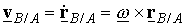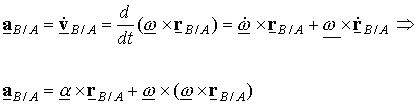# Rigid body relative acceleration

## Homework Statement

Rigid body (ship) rotate and moves around 3 axis (x,y,z) around the center of gravity. The position is of the center of gravity is not known.
What is known: At a point (A) the accereleration, velocity and position and rotational acceleration, velocity and position are known (measured). And the original position vector (relative to the fixed world) from point A to a point B.

What is wanted: The vertical (z-direction) displacement, velocity and acceleration of point B.

2. The attempt at a solution
With the known angles the relative vector (r_AB) between point A a B can be easily calculated with a rotation matrix:When you add the the measured position of A to this r_AB the new position of B is known.
Now the relative velocity can be calculated asso the velocity v_B=v_A+ v_B/A

and the relative acceleration is:So the acceleration is a_B=a_A+a_B/A.

Now the my first question is whether this is correct?
And secondly I find it strange that when you take the z-componont of the acceleration it is dependent on the rotational speed around the z-axis (because of the double cross product). Why is this? You would think this rotational speed around its axis has nothing to do with any movement or acceleration along that axis.

And also a similar problem with the velocity in z direction which is dependent on the yaw (the angle around the z axis). Which i also find strange.

Last edited by a moderator:

## Answers and Replies

Gold Member
I think it's because the z-axis is being rotated into the other axes that do change the z-axis acceleration. So the instantaneous (current) z-axis rotation will start changing the (moved) z-axis acceleration.

Last edited:
I think it's because the z-axis is being rotated into the other axes that do change the z-axis acceleration. So the instantaneous (current) z-axis rotation will start changing the (moved) z-axis acceleration.
But that would mean that the x,y and z axis are not fixed. And in my case i want that the axis are fixed to the world, because i want the know the position, velocity and acceleration of point B with regard to the ground (seabed). Does that mean i need another way to calculate my all this? Or did i misunderstood your explanation?